output.to from Sideway
Draft for Information Only

# Content

``` Matrices   Matrices   Square Matrix   Principal Diagonal   Trace   Vector   Zero Matrix  Equality of Matrices,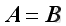```

# Matrices

## Matrices

A matrix, designated by a bold capital letter, is a collection of elements enclosed in brackets in the form of rectangular array with horizontal rows and vertical columns. A matrix is usually denoted by a upper case letter and an element of matrix is often denoted by a lower case letter with double subscript notation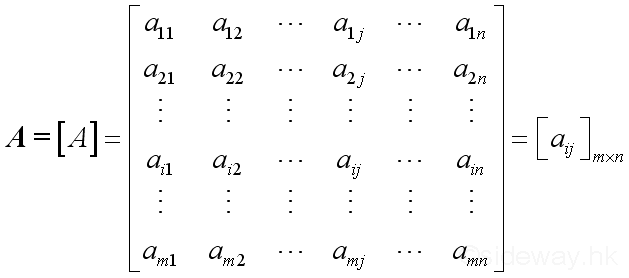The matrix A is of order m x n with m rows and n columns.

Row i of the matrix: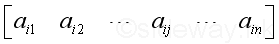;

Column j of the matrix: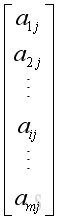Element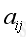is the matrix content at the intersection of row i and column j.

## Square Matrix

A matrix with the same number of rows as columns, m = n is called a square matrix of order n.

## Principal Diagonal

The elementswhere i = j forms the principal diagonal of a square matrix.

## Trace

The trace of a square matrix is the sum of the elements on the principal diagonal.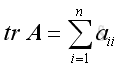## Vector

For a single row matrix, of order 1 x n, it is called a row vector or row matrix.

For a single column matrix, of order m x 1, it is called a column vector or column matrix.

A vector is usually represented by a lower case letter, e.g. a

## Zero Matrix

If a matrix of any order consists all elements zero, it is called a zero matrix or null matrix, O.

## Equality of Matrices,Let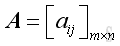and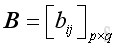,

if two matrices are of the same order,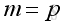&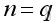and the corresponding elements are equal,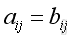, then two matrices are equal,ID: 100200007 Last Updated: 8/14/2010 Revision: 1Home 5

Management

HBR 3

Information

Recreation

Culture

Chinese 1097

English 337

Computer

Hardware 154

Software

Application 207

Latex 35

Manim 203

Numeric 19

Programming

Web 285

Unicode 504

HTML 65

CSS 63

SVG 9

ASP.NET 240

OS 422

Python 64

Knowledge

Mathematics

Algebra 84

Geometry 32

Calculus 67

Engineering

Mechanical

Rigid Bodies

Statics 92

Dynamics 37

Control

Natural Sciences

Electric 27